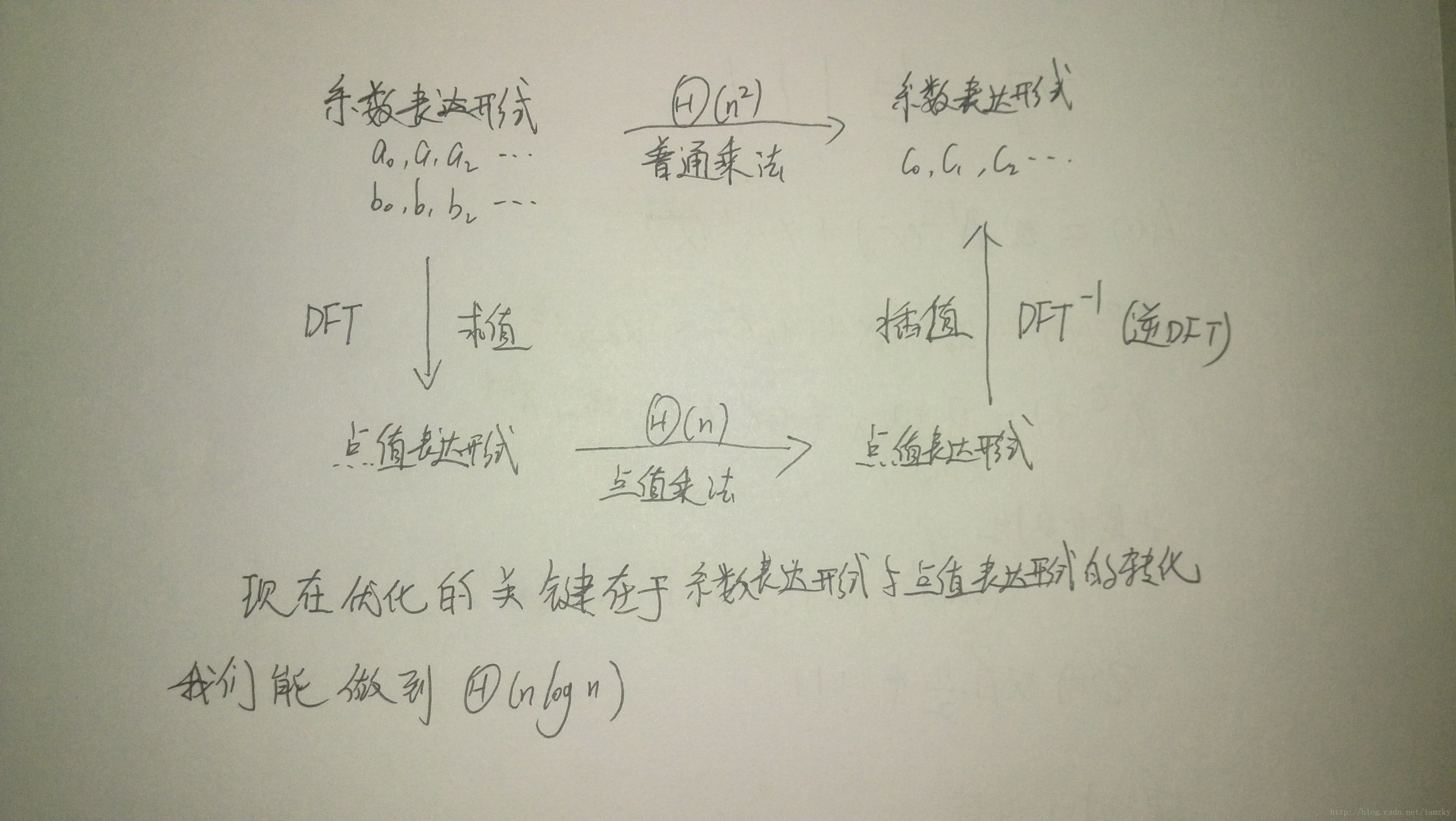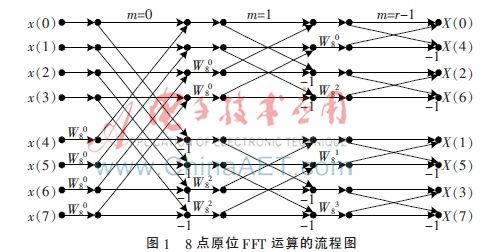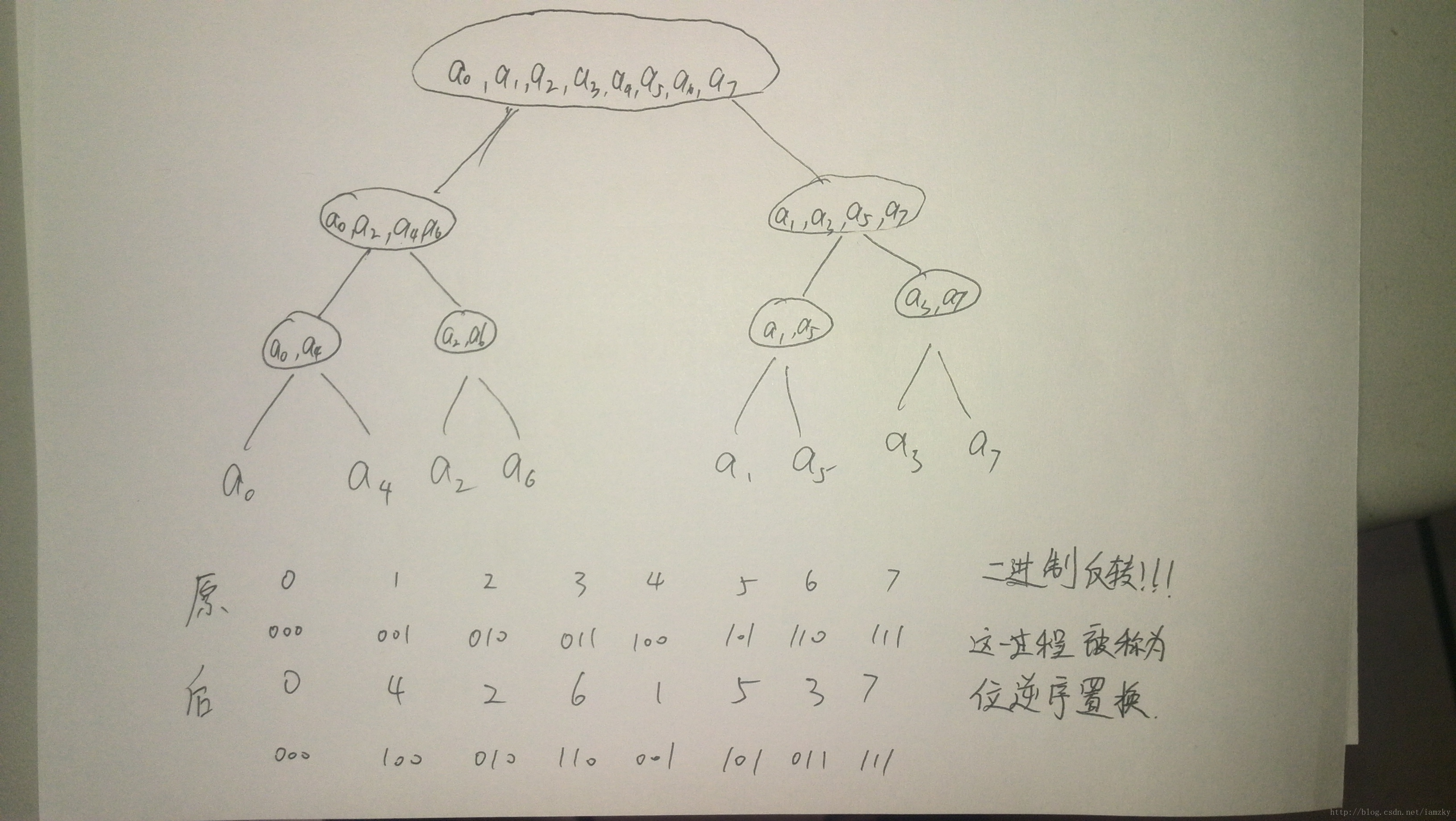# FFT [TPLY]

https://www.luogu.org/problemnew/show/1919
https://www.luogu.org/problemnew/show/3803

## 资料推荐

orz 大佬博客
http://www.cnblogs.com/cjoieryl/p/8206721.html (orz YL 大佬)
http://blog.csdn.net/iamzky/article/details/22712347 (超级易懂)

https://baike.baidu.com/item/%E5%8D%95%E4%BD%8D%E6%A0%B9

http://blog.csdn.net/acdreamers/article/details/39005227

http://blog.csdn.net/bitcarmanlee/article/details/54729807

ACM/ICPC 算法基础训练教程 7.4 快速傅里叶变换

# Part1. 初识 FFT

FFT 多项式乘法与普通形式有差异

FFT‘ 绕圈’ 法
FFT 处理多项式相乘, 先从多项式最普通的表达形式–-系数表达形式转化成点值表达形式再进行点值乘法. 最后, 再将点值乘法的结果转回系数表达形式.

１～对于点值表达式我的理解 :

２～点值表达式的计算:

A(x)={(0,1),(1,2),(2,7)}
B(x)={(0,2),(1,2),(2,4)}
[P.S.: 点乘表达式能运算的条件是两个点要有相同的 x 值]
C(x)={(0,2×1),(1,2×2),(2,7×4)}={(0,2),(1,4),(2,28)}

FFT 流程图如下:(借鉴他人博客)# part2. 复数

0. 为什么要学习复数?
YL 大佬原话:” 学习复数, 所有这些七七八八的定理, 都是为了 FFT 的奇妙变换!”

1. 复数的定义: 我们把形如 a+bi（a,b 均为实数）的数称为复数，其中 a 称为实部，b 称为虚部，i 称为虚数单位,i 的值为 $\sqrt{-1}$
2. 单位根的定义: 数学上，n 次单位根是 n 次幂为 1 的复数。

{$1$, $\frac{-1+\sqrt{3}i}{2}$,$\frac{-1-\sqrt{3}i}{2}$}

$(\frac{-1+\sqrt{3}i}{2})^3$=$(\frac{-1+\sqrt{3}i}{2})^2$×$(\frac{-1+\sqrt{3}i}{2})$
………………..=$(\frac{1+3i^2-2\sqrt{3}i}{4})$×$(\frac{-1+\sqrt{3}i}{2})$
………………..=$(\frac{-2-2\sqrt{3}i}{4})$×$(\frac{-1+\sqrt{3}i}{2})$
………………..=$(\frac{(-2)×(1+\sqrt{3}i)×(\sqrt{3}i-1)}{8})$
………………..=-$(\frac{1}{4})$×($3i^2-1$)
………………..=$-(\frac{1}{4})$*(-4)
………………..=1

PS 由于太弱不会证明,YL 大佬的博客上由详细证明可以参考
(w 上标表示指数)

$$\omega^{dk} _ {dn}=\omega^{k}_{n}$$

# Part3.DFT 与-DFT 进阶

DFT

$$y _ k=A(\omega^{k} _ {n}) = \Sigma^{n-1} _ {j=0} a _ j(\omega^{k} _ {j})^j$$

-DFT

$y _ k = \Sigma^{n-1} _ {j=0} a _ j(x)^j$

# Part4 FFT 水落石出

$$A^{}(x) = a _ 0 + a _ 2x + a_4x^2 + … + a _ {n-2}x^{\frac{n}{2} – 1}$$
$$A^{}(x) = a _ 1 + a _ 3x + a_5x^2 + … + a _ {n-1}x^{\frac{n}{2} – 1}$$
$$A(x) = A^{}(x^2) + xA^{}(x^2)$$

$$A(\omega^k _ n)=A^{}((\omega^k _ n)^2) + \omega^k _ n A^{}((\omega^k _ n)^2)$$

$（（\omega^k _ n)^2$=$\omega^{2k} _ n$

………….=$\omega^{\frac{2k}{2}} _ {\frac{n}{2}}$

………….=$\omega^{k} _ {\frac{n}{2}}$

$$\omega^{k+\frac{n}{2}} _ n=\omega^{k} _ n*\omega^{\frac{n}{2}} _ n=-\omega^{k} _ n$$

$$A(\omega^{k+\frac{n}{2}} _ n)=A^{}((\omega^{k+\frac{n}{2}} _ n)^2) + \omega^{k+\frac{n}{2}} _ nA^{}((\omega^{k+\frac{n}{2}} _ n)^2)$$
$$=A^{}((-\omega^k _ {n})^2)-\omega^k _ {n}A^{}((-\omega^k _ {n})^2)$$
$$=A^{}(\omega^{2k} _ {n})-\omega^k _ {n}A^{}(\omega^{2k} _ {n})$$
$$再一次用到咱们的消去引理 再一次用到咱们的消去引理$$
$$。。。（式）=A^{}(\omega^k _ {\frac{n}{2}})-\omega^k _ {n}A^{}(\omega^k _ {\frac{n}{2}})。。。（式 1）$$

$$A(\omega^k _ n)=A^{}((\omega^k _ n)^2) + \omega^k _ n A^{}((\omega^k _ n)^2)$$
$$=A^{}(\omega^{2k} _ n) + \omega^k _ n A^{}(\omega^{2k} _ n)$$
$$又因为消去引理 又因为消去引理$$
$$。。。（式）=A^{}(\omega^{k} _ {\frac{n}{2}}) + \omega^k _ n A^{}(\omega^k _ {\frac{n}{2}})。。。（式 2）$$

# 蝴蝶操作

$$A(\omega^k _ n)=A^{}(\omega^{k} _ {\frac{n}{2}}) + \omega^k _ n A^{}(\omega^k _ {\frac{n}{2}})$$
$$A(\omega^{k+\frac{n}{2}} _ n)=A^{}(\omega^k _ {\frac{n}{2}})-\omega^k _ {n}A^{}(\omega^k _ {\frac{n}{2}})$$4 -> 100
1 -> 001

（注意标号从 0 开始）

（链接再最上面）

# 代码

//P3803【模板】多项式乘法（FFT）
#include <algorithm>
#include <iostream>
#include <cstring>
#include <cstdio>
#include <queue>
#include <cmath>

#define rg register int
#define ll long long
#define db double
#define il inline

#define INF 2147483647
#define SZ 10000001

using namespace std;

const double pi=acos(-1);

struct Complex
{
db r,i;
Complex(){}
Complex(db a,db b):r(a),i(b) {}
Complex operator+(const Complex B)const
{return Complex(r+B.r,i+B.i);}
Complex operator-(const Complex B)const
{return Complex(r-B.r,i-B.i);}
Complex operator*(const Complex B)const
{return Complex(r*B.r-i*B.i,r*B.i+i*B.r);}
};
Complex X,Y,a[SZ],b[SZ];

int r[SZ],n,m,l;
il void FFT(Complex *a,int x)
{
for(rg i=0;i<n;++i) if(i<r[i]) swap(a[i],a[r[i]]);
for(rg i=1;i<n;i<<=1)
{
Complex wn(cos(pi/i),x*sin(pi/i));
for(rg j=0;j<n;j+=(i<<1))
{
Complex w(1,0);
for(rg k=0;k<i;++k,w=w*wn)
{
X=a[j+k],Y=a[i+j+k]*w;
a[j+k]=X+Y,a[i+j+k]=X-Y;
}
}
}
if(x==-1) for(rg i=0;i<n;++i) a[i].r=a[i].r/n;
}

int main()
{
scanf("%d%d",&n,&m);
for(rg i=0;i<=n;++i) scanf("%lf",&a[i].r);
for(rg i=0;i<=m;++i) scanf("%lf",&b[i].r);
m+=n;for(n=1;n<=m;n<<=1) ++l;
for(rg i=0;i<n;++i) r[i]=(r[i>>1]>>1)|((i&1)<<(l-1));
FFT(a,1);FFT(b,1);
for(rg i=0;i<=n;++i) a[i]=a[i]*b[i];
FFT(a,-1);
for(rg i=0;i<=m;++i) printf("%d ",(int)(a[i].r+0.5));
return 0;
}

P1919【模板】A*B Problem 升级版（FFT 快速傅里叶）
#include <algorithm>
#include <iostream>
#include <cstring>
#include <cstdio>
#include <queue>
#include <cmath>

#define rg register int
#define ll long long
#define il inline
#define ldb long double

#define INF 2147483647
#define SZ 1000001

using namespace std;
const ldb pi=acos(-1);

struct Complex
{
ldb r,i;
Complex(){}
Complex(ldb a,ldb b):r(a),i(b){}
il Complex operator+(const Complex B)const
{return Complex(r+B.r,i+B.i);}
il Complex operator-(const Complex B)const
{return Complex(r-B.r,i-B.i);}
il Complex operator*(const Complex B)const
{return Complex(r*B.r-i*B.i,r*B.i+i*B.r);}
};
Complex X,Y,a[SZ],b[SZ];
int n,m,l,r[SZ];
il void FFT(Complex *a,int x)
{
for(rg i=0;i<n;++i) if(i<r[i]) swap(a[i],a[r[i]]);
for(rg i=1;i<n;i<<=1)
{
Complex wn(cos(pi/i),x*sin(pi/i));
for(rg j=0;j<n;j+=(i<<1))
{
Complex w(1,0);
for(rg k=0;k<i;++k,w=w*wn)
{
X=a[j+k],Y=a[i+j+k]*w;
a[j+k]=X+Y,a[i+j+k]=X-Y;
}
}
}
if(x==-1) for(rg i=0;i<n;++i) a[i].r=a[i].r/n;
}

char ch1[SZ],ch2[SZ];
int res[SZ];
int main()
{
scanf("%d",&n);
cin>>ch1;
cin>>ch2;
for(rg i=0;i<n;++i)
{
a[i].r=ch1[n-i-1]-'0';
b[i].r=ch2[n-i-1]-'0';
}
m=n+n-2;for(n=1;n<=m;n<<=1) ++l;
for(rg i=0;i<n;++i) r[i]=(r[i>>1]>>1)|((i&1)<<(l-1));
FFT(a,1);FFT(b,1);
for(rg i=0;i<=n;++i) a[i]=a[i]*b[i];
FFT(a,-1);
for(rg i=0;i<=m;++i) res[i]=(int)(a[i].r+0.5);
for(rg i=0;i<=m;++i) res[i+1]+=res[i]/10,res[i]%=10;
rg top=m+1;
while(res[top]>9)
{
res[top+1]=res[top]/10;
res[top]%=10;
++top;
}
while(!res[top]) --top;
for(rg i=top;i>=0;--i)
printf("%d",res[i]);
return 0;
}


pi 最好不要手写（当然如果你能背到小数点后十几位我也不栏你）

### 5 条评论#### konnyakuxzy · 2018年1月13日 3:24 下午

QvQ 终于改完了，先 OrzLTP

latex 中可以用

$\times$


QvQ